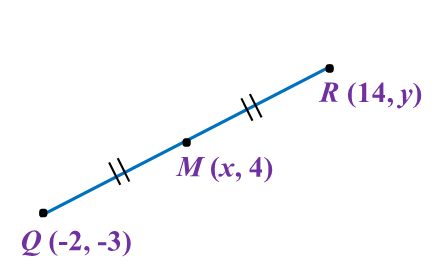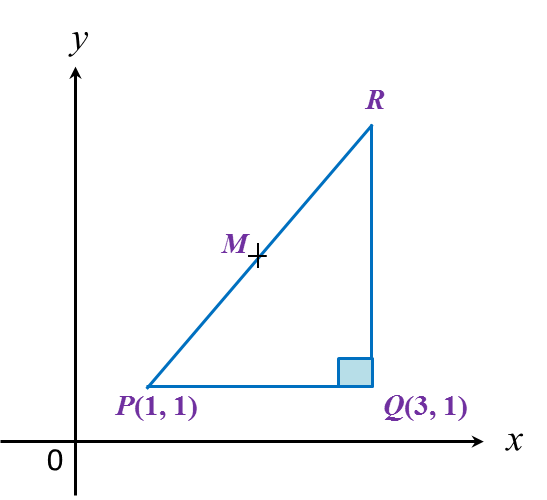# 7.2.2 Coordinates, PT3 Focus Practice

Question 4:
The point M (x, 4), is the midpoint of the line joining straight line Q (-2, -3) and R (14, y).The value of x and y are

Solution:

$\begin{array}{l}x=\frac{-2+14}{2}\\ x=\frac{12}{2}\\ x=6\\ \\ 4=\frac{-3+y}{2}\\ 8=-3+y\\ y=11\end{array}$

Question 5:

In diagram below, PQR is a right-angled triangle. The sides QR and PQ are parallel to the y-axis and the x-axis respectively. The length of QR = 6 units.Given that M is the midpoint of PR, then the coordinates of M are

Solution:

x-coordinate of R = 3
y-coordinate of R = 1 + 6 = 7
R = (3, 7)

Question 6:

Given points (–2, 8) and (10, 8), find the length of PQ.

Solution:

### 2 thoughts on “7.2.2 Coordinates, PT3 Focus Practice”

1.theres error in question 6, should be 12square then 12 units

•Thanks for pointing out our mistake.
We had done the correction accordingly.